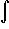#Interactive Real Analysis

Next | Previous | Glossary | Map

## 7.2. Integration Techniques

### Example 7.2.4(a): Applying the Substitution Rule

Here is a simple example: find(4x + 3)2 dx.
The expression that makes the integral difficult is 4x + 3 so that we make the change of variable
u = 4x + 3
Then
du/dx = 4, or du = 4 dx
which at first glance does not appear in the original integral. But:(4x + 3)2 dx =u2 1/4 4 dx =
= 1/4u2 4 dx = 1/4u2 du =
= 1/4 1/3 [(4b + 3)3 - (4a + 3)3]
There is no "incorrect" substitution, you can make any change of variables that you want. There are, however, substitutions that work (such as above) and those that do not work (which are not incorrect but not successful). For example, we could decide to make the following substitution in the above example:
u = (4x + 3)2
Then
du/dx = 2 (4x + 3) 4 or du = 8 (4x + 3) dx
The new integral looks likeu dx, but it is not (easily) possible to remove the dx. Therefore, this substitution, while correct, was not successful.
Next | Previous | Glossary | Map